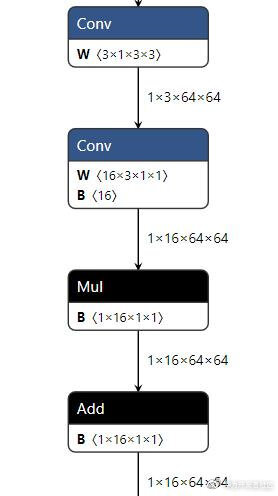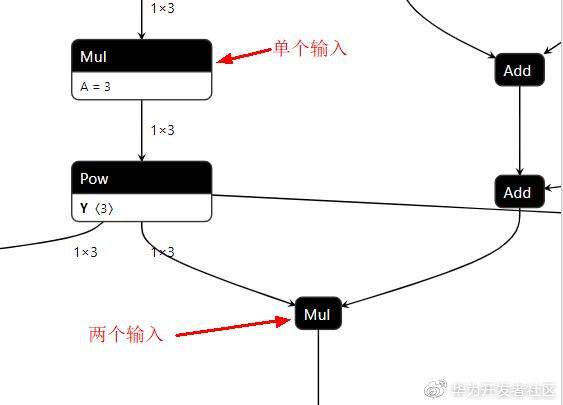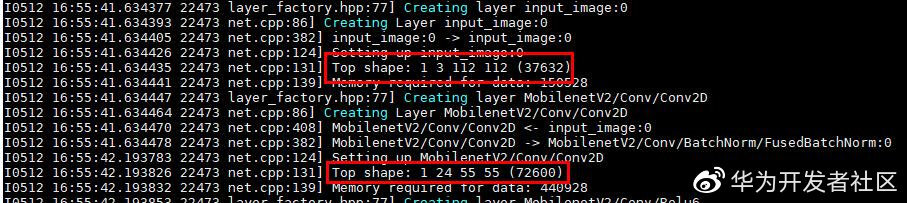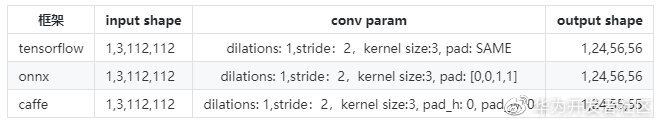# 将模型转为 NNIE 框架支持的 wk 模型第一步：tensorflow-＞caffe## tensorflow->caffe

python -m tf2onnx.convert --input ./model.pb --inputs input_image:0[1,112,112,3] --inputs-as-nchw input_image:0 --outputs output_0:0,output_1:0,output_2:0,output_3:0,output_4:0 --output ./convert.onnx

​得到 onnx 模型之后，可以使用onnx simplifer将一些零散算子合并，或者将一些冗余算子去除，这个工具视情况使用。

python -m onnxsim input_onnx_model output_onnx_model

onnx2caffe+-- onnx2caffe|   +-- _operators.py|   +-- _weightloader.py+-- convertCaffe.py+-- MyCaffe.py

​运行命令：

python convertCaffe.py ./model/MobileNetV2.onnx ./model/MobileNetV2.prototxt ./model/MobileNetV2.caffemodel

（1）遇到 caffe 与 NNIE 不支持的算子，可以修改 onnx 模型中的 node 以适配 caffe（这里要发动自己的小脑筋，一些算子替换可以参考一下pytorch->caffe这篇博客）。

（2）如果遇到了 NNIE 与 onnx 支持的算子，但是 caffe 1.0 官方不支持的话，可以在 caffe 中添加新的层，重新编译之后，再做转换。caffe 中添加新的层可以参考：caffe 添加新node

（3）caffe 与 NNIE 都支持的算子，但是转换工具没有支持该算子的转换，在转换代码中添加相应的算子实现。

（4）转换过程中算子转换成功，但是出现了 shape 问题，手动添加一些不需要参数的操作在已经生成的 prototxt 中。

## 修改 onnx 模型中的 node 以适配 caffe

onnx 支持的 op：onnx op

1.    关于 node 的改写有时需要已知其输入输出 size,故一开始先准备一个包含每个 node 输入输出的 onnx 模型。

import onnx.helper as helperfrom onnx import shape_inference, TensorProtoimport onnxruntimeimport onnxdef add_input_output_from_onnx(onnx_path, save_path):    ONNX_DTYPE = {        0: TensorProto.FLOAT,        1: TensorProto.FLOAT,        2: TensorProto.UINT8,        3: TensorProto.INT8,        4: TensorProto.UINT16,        5: TensorProto.INT16,        6: TensorProto.INT32,        7: TensorProto.INT64,        8: TensorProto.STRING,        9: TensorProto.BOOL    }    # load model    onnx_model = onnx.load(onnx_path)    graph = onnx_model.graph    # rewrite the input tensor of graph    input_tensor = graph.input    input_shape = input_tensor.type.tensor_type.shape.dim    input_tensor_new = onnx.helper.make_tensor_value_info(name = input_tensor.name, elem_type = 1,                                                           shape = [1, input_shape.dim_value, input_shape.dim_value, input_shape.dim_value])    graph.input.remove(input_tensor)    graph.input.insert(0, input_tensor_new)    # append all tensor infos to graph input    weight_infos = []    tensors = graph.initializer    for i, tensor in enumerate(tensors):        value_info = helper.make_tensor_value_info(tensor.name, ONNX_DTYPE[tensor.data_type], tensor.dims)        weight_infos.append(value_info)        graph.input.insert(i+1, value_info) # because 0 is for placeholder, so start index is 1    # run node shape inference    node = graph.node    value_info = graph.value_info    inferred_onnx_model = shape_inference.infer_shapes(onnx_model)    onnx.checker.check_model(onnx_model)    inferred_graph = inferred_onnx_model.graph    inferred_value_info = inferred_graph.value_info    onnx.save(inferred_onnx_model,save_path)    return​2.  遇到 caffe 与 NNIE 不支持的算子，删除 onnx 模型中的 node，将相关操作在外部的预处理阶段进行。这种情况只涉及 onnx 模型中已经存在的节点删除与改变已有边连接的关系，不涉及新的边关系的建立。

 这里使用graph中node的index来访问node  该代码删除graph node 0,1,2  并且修改node 3的input边  即   input_image --> mul_1 --> sub --> mul --> conv1  变为 input_image --> conv1def delete_node(onnx_path, save_path):    onnx_model = onnx.load(onnx_path)    graph = onnx_model.graph    Mul_1 = graph.node    sub = graph.node    mul = graph.node    conv1 = graph.node    conv1.input = Mul_1.input    graph.node.remove(Mul_1)    graph.node.remove(sub)    graph.node.remove(mul)    onnx.checker.check_model(onnx_model)    onnx.save(onnx_model, save_path)

​3.    更改 caffe 与 NNIE 不支持的算子，修改 onnx 模型中的 node 去适配。如 squeeze 算子，squeeze 算子在 onnx->caffe 的时候会报错，这时可以将 onnx 模型中的 squeeze 替换为 reshape 算子。reshape 需要两个输入，而 squeeze 只对应一个输入，这时需要在 graph 中创建一个新的常数 tensor input。这种情况涉及更换已经存在的 node，新的常数 tensor 的加入，但并不涉及新的边关系的建立。

查看onnx op的操作，reshape需要两个输入对于reshape需要将一个shape tensor加入到onnx graph中，tensor size可以查看第一步生成的onnx model中该squeeze node对应的output size即   input --> squeeze --> output变为 input --> reshape(shape) --> outputdef remove_headpose_squeeze_node(onnx_path, save_path):    onnx_model = onnx.load(onnx_path)    graph = onnx_model.graph    ## 添加常数 input    shape = onnx.helper.make_tensor('shape', onnx.TensorProto.INT64, , [1,3])    graph.initializer.append(shape)    for i in range(len(graph.node)):        if graph.node[i].op_type == "Squeeze":            reshape_node_def = helper.make_node(                        'Reshape', # node name                        inputs=[graph.node[i].input, 'shape'], # inputs                        outputs=[graph.node[i].output], # outputs                        name = graph.node[i].name                    )            graph.node.remove(graph.node[i])            graph.node.insert(i, reshape_node_def)    onnx.checker.check_model(onnx_model)    onnx.save(onnx_model, save_path)

​4.    caffe 不支持 div 算子，可以将 div 算子转为 pow+mul。这种情况涉及将一个 node 更换为两个，新的常数 tensor 的加入，以及新的边连接关系。

div 操作： z = x / y

temp = pow(y, -1)

z = temp * x

即：input_x    input_y \\   //  \\ //   div更改为：input_x         input_y \\         //  \\       //   \\      pow（常数tensor作为指数输入）    \\    //     \\  //  --> （新的边）      muldef change_headpose_div_node(onnx_path, save_path):    onnx_model = onnx.load(onnx_path)    graph = onnx_model.graph    pow_scale = onnx.helper.make_tensor('pow_scale', onnx.TensorProto.FLOAT, , [-1.0, -1.0, -1.0])    mul12_output = helper.make_tensor_value_info('pred_pose/mul_12_pow_output:0', onnx.TensorProto.FLOAT, [1, 3])    graph.initializer.append(pow_scale)   # 'pred_pose/mul_12:0' 类似于上图中的input_y   #  pow_scale 为上面创建的相应的指数tensor   # 'pred_pose/mul_12_pow_output:0' 为新建的output tensor   #  pow name 给一个不与图中node重复的name    mul12_pow_node_def = helper.make_node(        'Pow', # node name        inputs=['pred_pose/mul_12:0', 'pow_scale'], # inputs        outputs=['pred_pose/mul_12_pow_output:0'], # outputs        name = 'pred_pose/mul_12_pow'    )    graph.node.insert(len(graph.node), mul12_pow_node_def)    for i in range(len(graph.node)):        if graph.node[i].name == "pred_pose/truediv_3":            input1 = graph.node[i].input            input2 = graph.node[i].input            output = graph.node[i].output            name = graph.node[i].name            pow_node_def = helper.make_node(                'Mul', # node name                inputs=[input1, mul12_pow_node_def.output], # inputs                outputs=[output], # outputs                name = name            )            print(graph.node[i].name, i)            graph.node.remove(graph.node[i])            graph.node.insert(i, pow_node_def)            break    graph = helper.make_graph(graph.node, graph.name, graph.input, graph.output, graph.initializer)    info_model = helper.make_model(graph)    model = onnx.shape_inference.infer_shapes(info_model)    onnx.save(model, save_path)

​经过这个修改之后，使用 netron 查看 node 边关系，看是否正确。

5.    打印 onnx 中间某个节点的输出，需要在 graph 加一个 output tensor。

def add_outputNode_info(onnx_path, add_name, output_size, save_path):    onnx_model = onnx.load(onnx_path)    graph = onnx_model.graph    prob_info =  helper.make_tensor_value_info(add_name,onnx.TensorProto.FLOAT, output_size)    graph.output.insert(0, prob_info)    onnx.save(onnx_model, save_path)    returnif __name__ == '__main__':    onnx_model = './model.onnx'    add_node_path = "./addPreprocessOutput.onnx"    # "mul:0": 想要输出node的output name    # [1,24,14,14]: 想要输出node的output size    add_outputNode_info(onnx_model, "mul:0", [1,24,14,14], add_node_path)

​上面的例子已经将大部分 node 修改的情况涵盖了，修改 onnx 模型可以参考上述代码。

## 在转换代码中添加相应的算子实现

1.    caffe 和 NNIE 都支持某个操作，但是 onnx2caffe 模型转换时报错。

_operators.py 文件用来实现 onnx 操作到 Caffe 操作的变换。对于 TanH 的适配，首先需要在文件的最后注册算子模块添加 TanH，然后增加转换代码。

转换代码：def _convert_tanH(node,graph,err):    input_name = str(node.inputs)    output_name = str(node.outputs)    name = str(node.name)    layer = myf("TanH",name,[input_name],[output_name])    graph.channel_dims[output_name] = graph.channel_dims[input_name]    return layer    添加注册算子：_ONNX_NODE_REGISTRY = {    ……    "Tanh": _convert_tanH,}

​_weightloader.py 文件用来实现 node 参数从 onnx 到 Caffe 的传递。第一步也是在文件末尾添加注册算子，添加同_operators.py。第二步，从 caffe.proto 中查看 tanh 操作是否存在 weight：

message TanHParameter {  enum Engine {    DEFAULT = 0;    CAFFE = 1;    CUDNN = 2;  }  optional Engine engine = 1 [default = DEFAULT];}

def _convert_tanH(net, node, graph, err):    pass

caffe 和 NNIE 都支持某个操作，onnx2caffe 也支持该操作，但是操作中有一个输入在模型中被写为 weight，与原来的实现不一致。​这种情况下，由于已经存在了 mul 的注册算子，我们只需要在 mul 算子转换的时候新加一个分支来实现就可以了，还是只涉及两个文件的改写。

_operators.py 添加分支代码

def _convert_input1_is_weight_mul(node,graph,max_dim, err):    node_name = node.name    这里的input_name需要在netron视图中观察一下是哪一个input作为外部输入，这里不能写 weight 的输入名称！    input_name = str(node.inputs)    output_name = str(node.outputs)    scale_layer = myf("Scale", node_name, [input_name],[output_name],in_place=False,bias_term=False)    graph.channel_dims[output_name] = max_dim    return scale_layerdef _convert_Mul(node,graph,err):    input_name_list = [str(i) for i in node.inputs]    output_name = str(node.outputs)    node_name = node.name    这里使用node_name 判断mul算子是否是一个input，新增只有一个input的分支    if node_name == "mul_1":        max_dim = 16        return _convert_input1_is_weight_mul(node,graph,max_dim, err)    ···    ···

def _convert_input1_is_weight_mul(net, node, graph, err):    node_name = node.name     注意！！       scale = np.ones(3) * 3.0       对应的是 外部输入size =（1,3）， weight size = (1),      这种情况可以借助 numpy 实现weight与外部输入的channel对齐      这里还有另外一种情况，例如 外部输入 size = (1,128,8,8), weight = (1,128,1,1)      可以这样操作：scale = node.input_tensors[node.inputs]                   scale = np.reshape(scale, scale.shape)         scale = np.ones(3) * 3.0    np.copyto(net.params[node_name].data, scale, casting='same_kind')mul本身是没有weight的，所以之前就是直接passdef _convert_Mul(net, node, graph, err):    node_name = node.name    if node_name == "mul_1":        _convert_input1_is_weight_mul(net, node, graph, err)    else：       pass

​实际转换过程中，add 算子也会出现上面的情况，其中有一个输入作为算子参数，这时可以把其类比到 _convert_BatchNorm 中的 scale 操作，将 scale 的 weight 视为 1，bias 为 add 算子的内部输入参数，可以参照 BatchNorm 修改代码，这里就不详细写了。

## 转换过程中算子转换成功，但是出现了 shape 问题,手动修改 prototxt​知己知彼方能百战百胜，为了定位 shape 为什么不一致，我们先要了解一下不同框架的 padding 策略以及相应的 output size 的计算方法。

• 查看 caffe 的 output size 计算方式，根据代码可得:

template <typename Dtype>void ConvolutionLayer<Dtype>::compute_output_shape() {    const int* kernel_shape_data = this->kernel_shape_.cpu_data();    const int* stride_data = this->stride_.cpu_data();    const int* pad_data = this->pad_.cpu_data();    const int* dilation_data = this->dilation_.cpu_data();    this->output_shape_.clear();    for (int i = 0; i < this->num_spatial_axes_; ++i) {      // i + 1 to skip channel axis      const int input_dim = this->input_shape(i + 1);      const int kernel_extent = dilation_data[i] * (kernel_shape_data[i] - 1) + 1;      const int output_dim = (input_dim + 2 * pad_data[i] - kernel_extent)/ stride_data[i] + 1;      this->output_shape_.push_back(output_dim);    }}

• tensorflow 的 padding 策略可根据这篇博客,结合上面 caffe 的 outputsize 计算，感觉 caffe 的 conv padding 策略与 tensorflow pad=VALID 一致，会把不能参与的 pixel 自动去除不进行计算。​分析上面模型转换的表格参数：

• tensorflow pad=SAME，为了使所有的 input pixel 都参与计算，tensorflow 在推理时偷偷在 input 的右下补了一行 0，这样最后的输出：

output size = (112 - (1 * (3 - 1) + 1) + 1) / 2 + 1 = 56

• 对于 onnx，经过查询与实验，发现 pads 参数[0,0,1,1]表示 feature map 上面不补，左边不补，下面补一行 0，右边补一列，与 tf 一致，输出没有什么问题。

• 转为 caffe 之后，caffe 模型 conv pad 参数都为 0，上下左右都不补，这时根据 caffe 的 outputshape 公式，最终计算结果为（1,3,55,55），直接去除 input 的最后一行和最后一列不参与计算。

caffe 中设置 pad_h:2, pad_w:2。 由于 caffe 是设置 pad 参数之后是对称补 0 的，即 input 的上下左右都补了两行或者两列 0，这时结合 output_shape 公式，最终输出的 shape 为：

output_shape = floor((112 + 2 * 2 - (1 *(3 - 1) + 1) + 1) / 2) + 1 = 57

layer {  name: "add_slice1"  type: "Slice"  bottom: "depthwise:0"  top: "add_slice1/split:0"  top: "add_slice1/split:1"  slice_param {    axis: 2    slice_point: 1  }}layer {  name: "add_slice2"  type: "Slice"  bottom: "add_slice1/split:1"  top: "add_slice2/split:0"  top: "add_slice2/split:1"  slice_param {    axis: 3    slice_point: 1  }}

​上面就是针对 caffe 模型的适配，东西很多很杂，有时候需要一些新奇的思路才能解决问题，当然还涉及一些 prototxt 文件中算子 param 的修改，具体问题具体分析，这里就不展开讲了。

## 总结## 评论